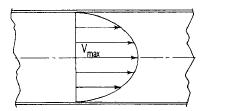Search

# Fluid flow in pipes

Posted in Hydraulics |Email This Post |

Laminar Flow

Its is a flow in which the fluid particles move in parallel layers in a single direction.Due to the parabolic velocity distribution in laminar flow, a shearing stress is developed. As this shearing stress increases, the viscous forces become unable to damp out disturbances, and turbulent flow results. The region of change is dependent on the fluid velocity, density, and viscosity and the size of the conduit.

Reynolds number which is the ratio of inertial forces/viscous forces is basically used to determine the kind of flow that is whether it is laminar, turbulent or is in transition .

Reynolds number <2000 ==laminar

Reynolds number >2000 == turbulent.

R=VD?\u=VD\v

where
V=fluid velocity,ft/s(m/s)
D=pipe Diameter,ft(m)
?=density of fluid,lb-s2/ft4
u=viscosity of fluid lb-s/ft2(kg-s/m2)
v=u/?=kinematic viscosity,ft2/s(m2/s)Diagram showing Laminar Flow

In laminar flow.the following equation for head loss due to friction can be developed by considering the forces acting on a cylinder of fluid in a pipe:

hf=32uLV/D2?g

where
L=length of pipe section considered,ft(m)
g=Acceleration due to gravity(9.81 m/s2)

Turbulent Flow

The inertial forces are large due to which the viscous forces cannot dampen out the disturbances which in turn create eddies.These eddies have a rotational and translational velocity.